# Methods and formulas for probabilities of missclassification in Crossed Gage R&R Study

Select the method or formula of your choice.

## Probabilities of misclassification

Minitab calculates the probabilities of misclassifications as both joint probabilities and conditional probabilities when you enter at least one specification limit.

### Joint probabilities

Probability that part is bad and you accept it: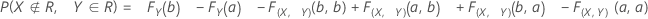Probability that part is good and you reject it: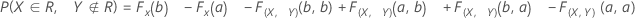### Conditional probabilities

Probability that given a part is bad, you accept it (false accept):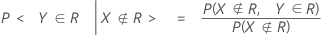Probability that given a part is good, you reject it (false reject):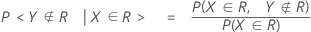### Notation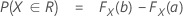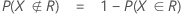F(X,Y) is the cumulative distribution function (CDF) of the bivariate normal random vector (X,Y)T with:

mean, μ = (θ,θ)T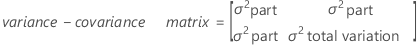F(X) and F(Y) are the corresponding marginal CDFs.

That is,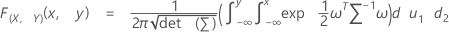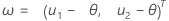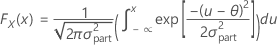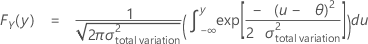By using this site you agree to the use of cookies for analytics and personalized content.  Read our policy